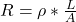## All other things being equal, current will be larger in a wire that has a larger value of All other things being equal, current will be larg

Question

All other things being equal, current will be larger in a wire that has a larger value of All other things being equal, current will be larger in a wire that has a larger value of:______

a. conductivity.
b. resistivity.
c. the coefficient of current net charge.
d. potential.

in progress 0
5 months 2021-09-05T16:13:41+00:00 1 Answers 12 views 0

a) conductivity

Explanation:

• Assuming the wire obeys Ohm’s Law, for a given voltage, in order to have a larger current, the resistance must be lower.
• The resistance of a resistive element is given by the following equation:• If geometric parameters keep the same, the only choice to lower the resistance value is diminishing the resistivity ρ.
• The resistivity, indeed, is defined as the inverse of the conductivity, σ.
• So, if resistivity decreases, conductivity increases.
• So, in order to have a larger current, all other things being equal, the wire must have a larger value of conductivity.
• The option a) is the right one.